# Difference between revisions of "Satake compactification"

A type of compactification arising from work of I. Satake on the compactification of quotients of symmetric spaces by arithmetically-defined groups ([a9], [a10], [a11]). Below, the simplest case of this is presented first, to help suggest its generalization.

Letbe the upper half-plane, the symmetric space of non-compact type for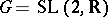. For any subgroupof finite index in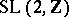— these are arithmetic groups (cf. also Arithmetic group) — the quotient space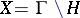is a Riemann surface, a modular curve. A compactification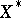ofis obtained by first taking the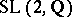-invariant set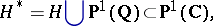with an-equivariant topology that is the given one onand makes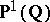discrete; a deleted neighbourhood base for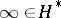is given byThenis taken to be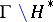. It is a compact Riemann surface (thus automatically an algebraic curve). An important ingredient, both here and in Satake's generalization, is reduction theory. Relative to the point, it asserts that ifand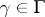satisfyand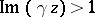, thenlies in the group of real translations (equivalently, in the parabolic groupof upper-triangular matrices, which is the stabilizer of). This gives an embedding of the punctured disc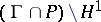in, and one is inserting the missing origin by adjoiningto.

There was great interest in doing something similar for, the moduli space of Abelian varieties (cf. also Moduli theory; Abelian variety), which is the quotient; here,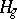is the Siegel upper half-space of genus, which is the symmetric space for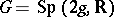, the rank-group of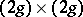symplectic matrices. Forone has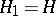(from the preceding paragraph). Satake first observed, in [a9], that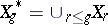could be topologized in a way that makes it a compact space with "hereditary" structure: the closure of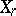inis homeomorphic to. Some refer to this space as "the" Satake compactification of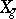.

Satake compactifications, in the sense of [a13] (after [a11] and [a3]), are defined from the following setting [a10]. Letbe the symmetric space of non-compact type for the real semi-simple Lie group(cf. also Lie group, semi-simple). Each faithful finite-dimensional representation of(cf. also Representation of a Lie algebra) determines an embedding ofin some real projective space, so let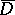be the closure of. The boundary ofconsists of pieces, called boundary components, that are homogeneous under a class of parabolic subgroups of. Ifis the (real) rank of, there are only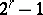distinct such spacesup to homeomorphism, corresponding to the non-empty subsetsof a set of simple roots, and as such they form a semi-lattice; if one then writes, the identity mapping ofextends to a continuous mappingwhenever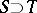.

Whenis the real Lie group associated to an algebraic group defined over, a boundary component is said to be rational when its normalizing parabolic subgroup is defined over. Likewise, the structure overdetermines the class of arithmetic subgroupsof. Take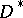to be the union ofand its rational boundary components. Then, with a suitable topology on,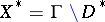is, under mild hypotheses, a compactification of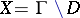. The collection of these inherit the semi-lattice structure from the above. There is a precise sense in which the topology ofis induced from that of the closure of a Siegel set in.

The Baily–Borel compactification is one of the minimal Satake compactifications in the case whereis Hermitian, i.e., has a-invariant complex structure (cf. also Hermitian symmetric space). This includes the case ofabove. Here,gets embedded as a bounded symmetric domain. By means of automorphic forms of sufficiently high weight,gets embedded as a normal algebraic subvariety of complex projective space [a2]. This fact has rather strong consequences, for the singular locus ofhas "high" codimension in general, and one can invoke general results from algebraic geometry. It implies the existence of big families of Abelian varieties that do not degenerate. This compactification also enters into the topological interpretation of the-cohomology of, as conjectured in [a12] and proved by E. Looijenga, and L. Saper and M. Stern.

The applications of Satake compactifications cover a range of other areas. The stable cohomology of "the" Satake compactification was determined by R. Charney and R. Lee [a5] for application in-theory. Non-Hermitian Satake compactifications occur in the combinatorial data of the toroidal compactifications of [a1] (see [a8], § 2), which provide resolution of the singularities of(cf. also Resolution of singularities).

Another compactification, the reductive Borel–Serre, a simple quotient of the manifold-with-corners constructed in [a4] (see, e.g., [a7]), dominates all Satake compactifications, and often coincides with the unique maximal one. It has played an increasing role in the theory of automorphic forms. It is the natural place to study the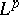-cohomology [a12] and to define weighted cohomology [a6].

The spaces(also defined in a different manner by H. Furstenberg) themselves have played an important role in harmonic analysis, rigidity theory and potential theory.

How to Cite This Entry:
Satake compactification. Encyclopedia of Mathematics. URL: http://encyclopediaofmath.org/index.php?title=Satake_compactification&oldid=16442
This article was adapted from an original article by Steven Zucker (originator), which appeared in Encyclopedia of Mathematics - ISBN 1402006098. See original article